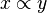Proportionality facts for kids

Kids Encyclopedia Facts

A proportionality relationship happens when two quantities or numbers x and y are related multiplicatively by a fixed number. This can occur when either their ratio x/y is a fixed number (direct proportionality), or their product xy is a fixed number (inverse proportionality). If x is directly proportional to y, then we write$x \propto y$. The fixed number of a proportionality relationship is called the constant of proportionality.

Related pagesProportionality Facts for Kids. Kiddle Encyclopedia.